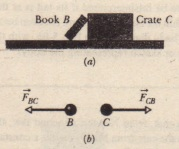# Newton’s Third Law

Newton’s Third Law

Two bodies are said to interact when they push or pull on each other that is, when a force acts on each body due to the other body. For example, suppose that you position a book B so it leans against a crate C . Then the book and crate interact: There is a horizontal force  on the book from the crate (or due to the crate) and a horizontal force  on the crate from the book (or due to the book). This pair of forces is shown in . Newton’s third law .

Where the minus sign means that these two forces are in opposite directions. We can call the forces between two interacting bodies a third law force pair. When any two bodies interact in any situation, a third-law force pair is present. The book and crate in  are stationary, but the third law would still hold if they were moving and even if they were accelerating.

As other example, let us find the third law force pairs involving the cantaloupe in lies on a table that stands on Earth. The cantaloupe interacts with the Earth (this time, there are three bodies whose interactions we must  out) .(a) Book B leans against crateC. (b) According to Newton’s thirdlaw, the force Fac on the book fromthe crate has the same magnitude butthe opposite direction of the force FCIIon the crate from the book(a) A cantaloupe lies on a table that stands on Earth. (b) The forces on the cantaloupeare Fa and FcE. (c) The third-law force pair for the cantaloupe-Earth interaction.(d) The third-law force pair for the cantaloupe-table interactionLet’s first focus only on the cantaloupe. Force  is the normal force on the cantaloupe from the table, and force is the gravitational force on the cantaloupe due to Earth. Are they a third-law force pair? No, they are forces on a single body, the cantaloupe, and not on two interacting bodies.

To find a third-law pair, we should focus not on the cantaloupe but on the
interaction between the cantaloupe and one of the other two bodies. First, in the cantaloupe- Earth interaction , Earth pulls on the cantaloupe with a gravitational force  and the cantaloupe pulls on Earth with a gravitational force F EC’ Are these forces a third-law force pair? Yes, they are forces on two interacting bodies, the force on each due to the other. Thus, by Newton’s third law,Next, in the cantaloupe-table interaction, the force on the cantaloupe from the table is F  and the force on the table from the cantaloupe .These for  also a third law force pair, and so.Suppose that the cantaloupe and table of  are in an elevator cab that begins to accelerate upward. (a) Do the magnitudes of forces and F in crease, decrease, or stay the same? (b) Are those two forces still equal in  magnitude and opposite in direction? (c) Do the magnitudes of forces FCE and FEc increase. decrease, or stay the same? (d) Are those two forces still equal in magnitude and opposite in direction?